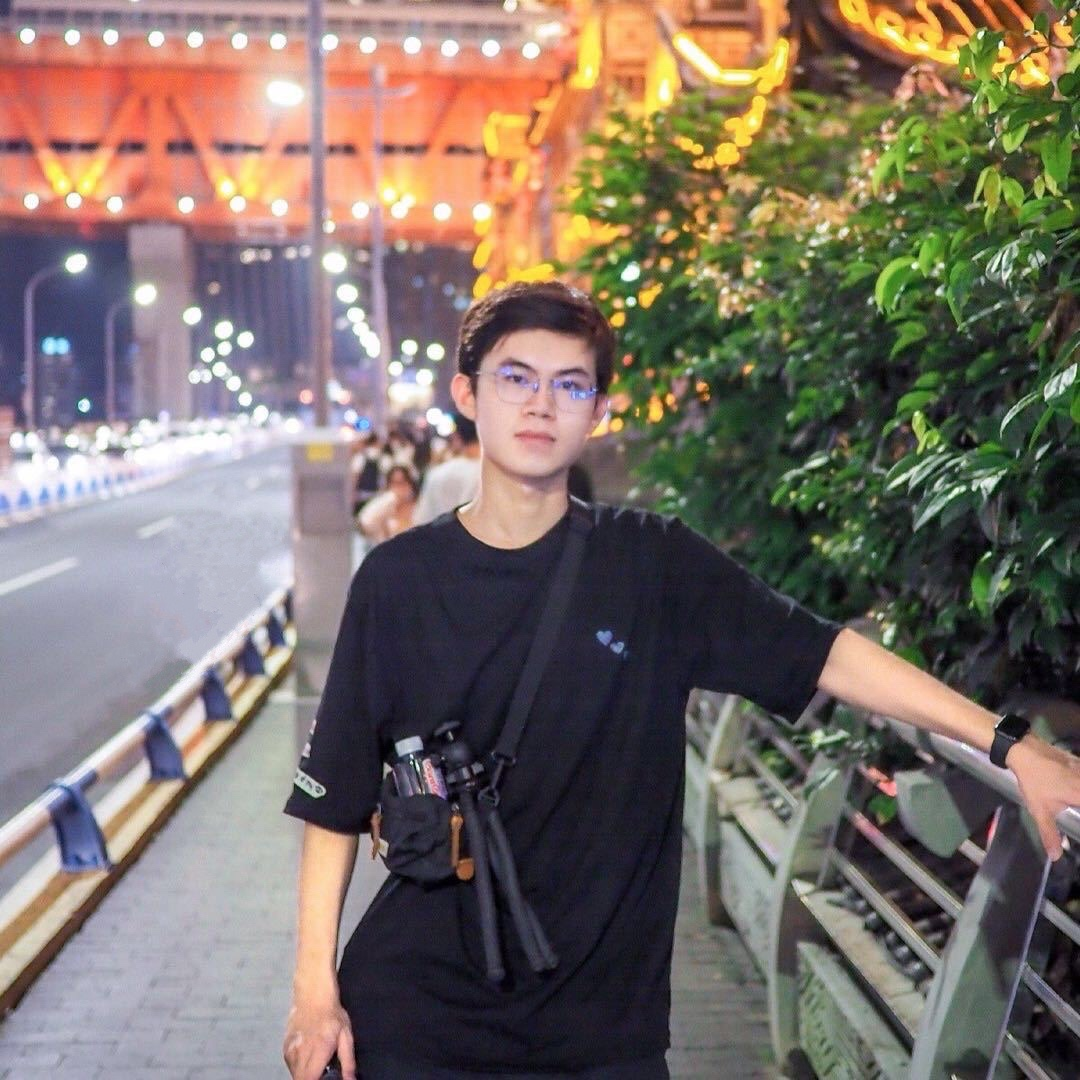cv_opse_estimateJinning Li Nov 15, 2017

# 2017.11.15

• CNN based method
• coordination regression
• heatmap
• stacked hourglass networks # 2017.11.22
• Scale
• openpose
• Town-down method
• recall 99%

• STN: spartial transform network
• $\theta$ automatic parameter for bounding box
• $\theta^{-1}$ reverse transformation
• Symmetric STN: this network can be lazy. to avoid STN not transform the pose to the center, parallel SPPE
• distribution error differ by the detection tools: fastRCNN, SSD, etc.
• RMPE CMUpose

• 3D pose